## 《概率论与数理统计》21春平时作业2

《概率论与数理统计》21春平时作业2

------------------------
1.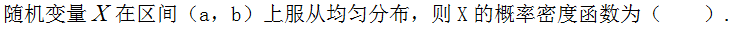A.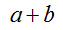B.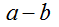C.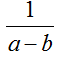D.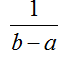2.设0，1，0，1，1来自X～0-1分布总体的样本观测值，且有P{X=1}=p，P{X=0}=q，其中0<p<1，q=1-p，则p的矩估计值为（ ）
A.1/5
B.2/5
C.4/5
D.3/5

3.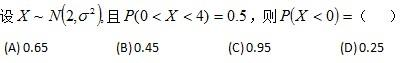A.（A）
B.（B）
C.（C）
D.（D）

4.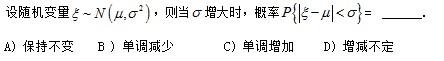A.（A）
B.（B）
C.（C）
D.（D）

5.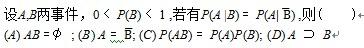A.（A）
B.（B）
C.（C）
D.（D）

6.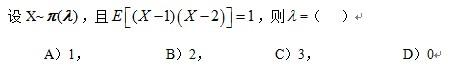A.（A）
B.（B）
C.（C）
D.（D）

7.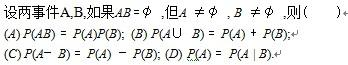A.（A）
B.（B）
C.（C）
D.（D）

8.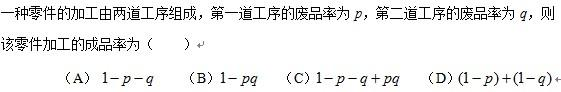A.（A）
B.（B）
C.（C）
D.（D）

9.图片1-17
A.（A)
B.(B)
C.(C )
D.(D)

10.图片2-4
A.（A)
B.(B)
C.(C )
D.(D)

11.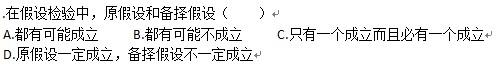A.（A）
B.（B）
C.（C）
D.（D）

12.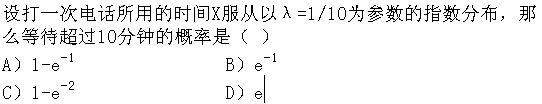A.（A）
B.（B）
C.（C）
D.（D）

13.图片3-12
A.（A)
B.(B)
C.(C )
D.(D)

14.设某试验成功的概率为p，独立地做5次该试验，成功3次的概率为（ ）
A.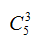B.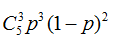C.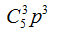D.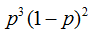15.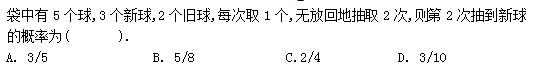A.（A）
B.（B）
C.（C）
D.（D）

16.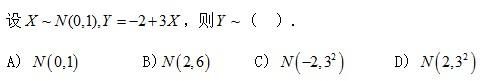A.（A）
B.（B）
C.（C）
D.（D）

17.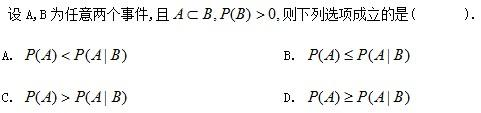A.（A）
B.（B）
C.（C）
D.（D）

18.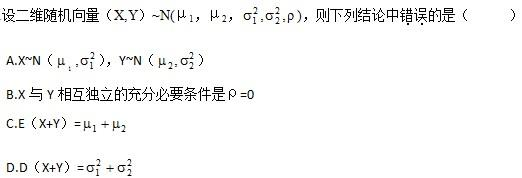A.（A）
B.（B）
C.（C）
D.（D）

19.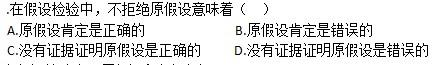A.（A）
B.（B）
C.（C）
D.（D）

20.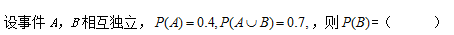A.0.2
B.0.3
C.0.4
D.0.5

------------------------
1.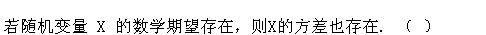A.正确
B.错误

2.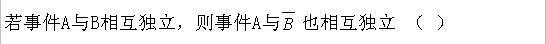A.正确
B.错误

3.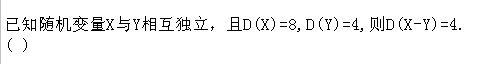A.正确
B.错误

4.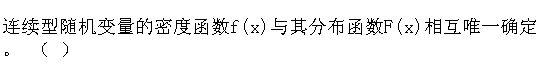A.正确
B.错误

5.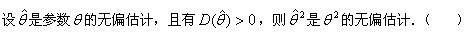A.正确
B.错误

6.图片1-23
A.错误
B.正确

7.图片2-22
A.错误
B.正确

8.图片1-30
A.错误
B.正确

9.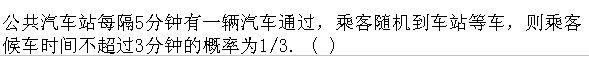A.正确
B.错误

10.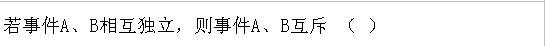A.正确
B.错误